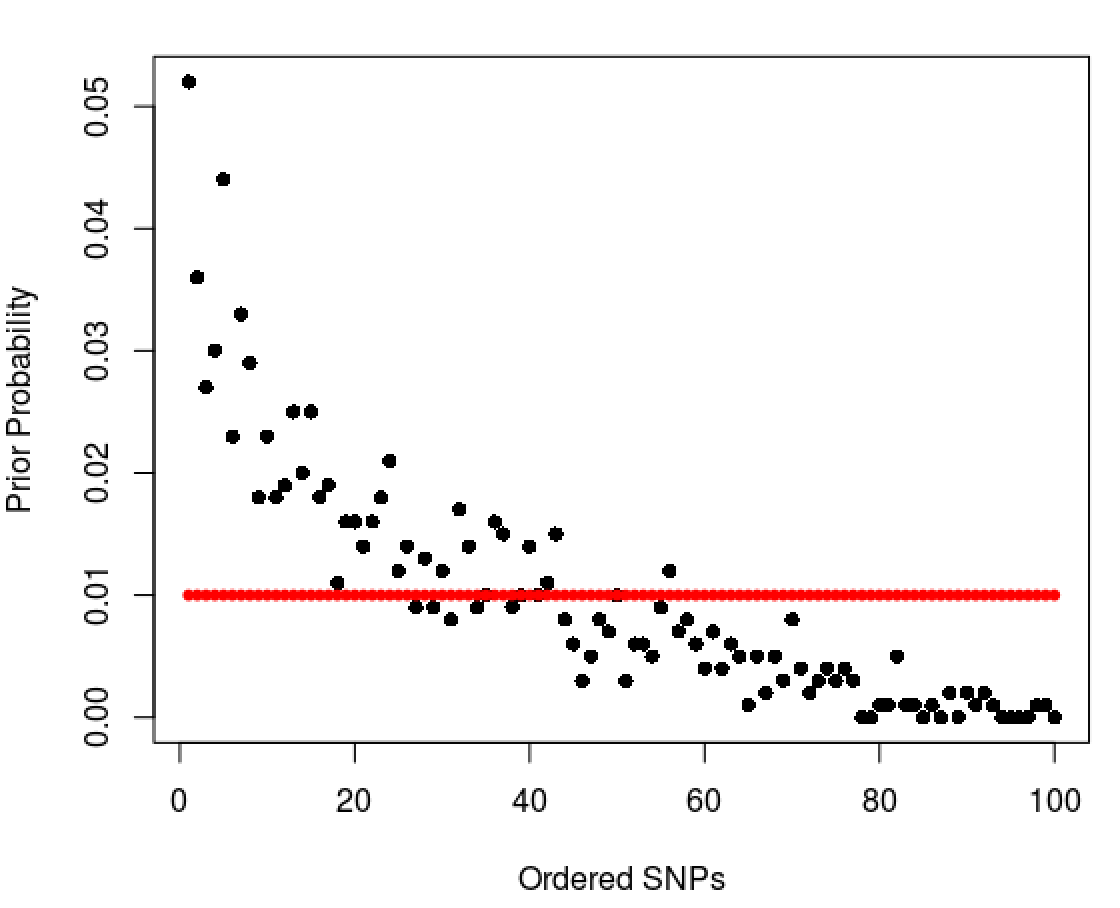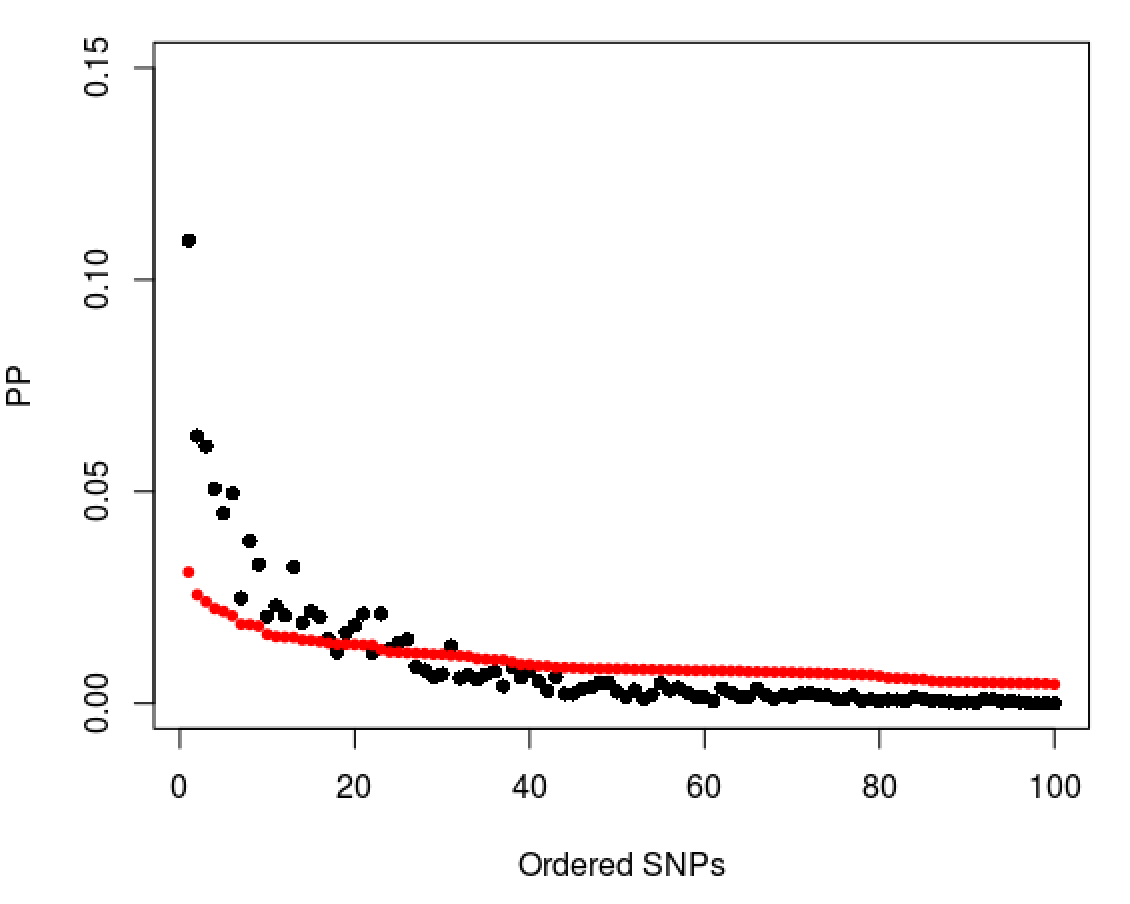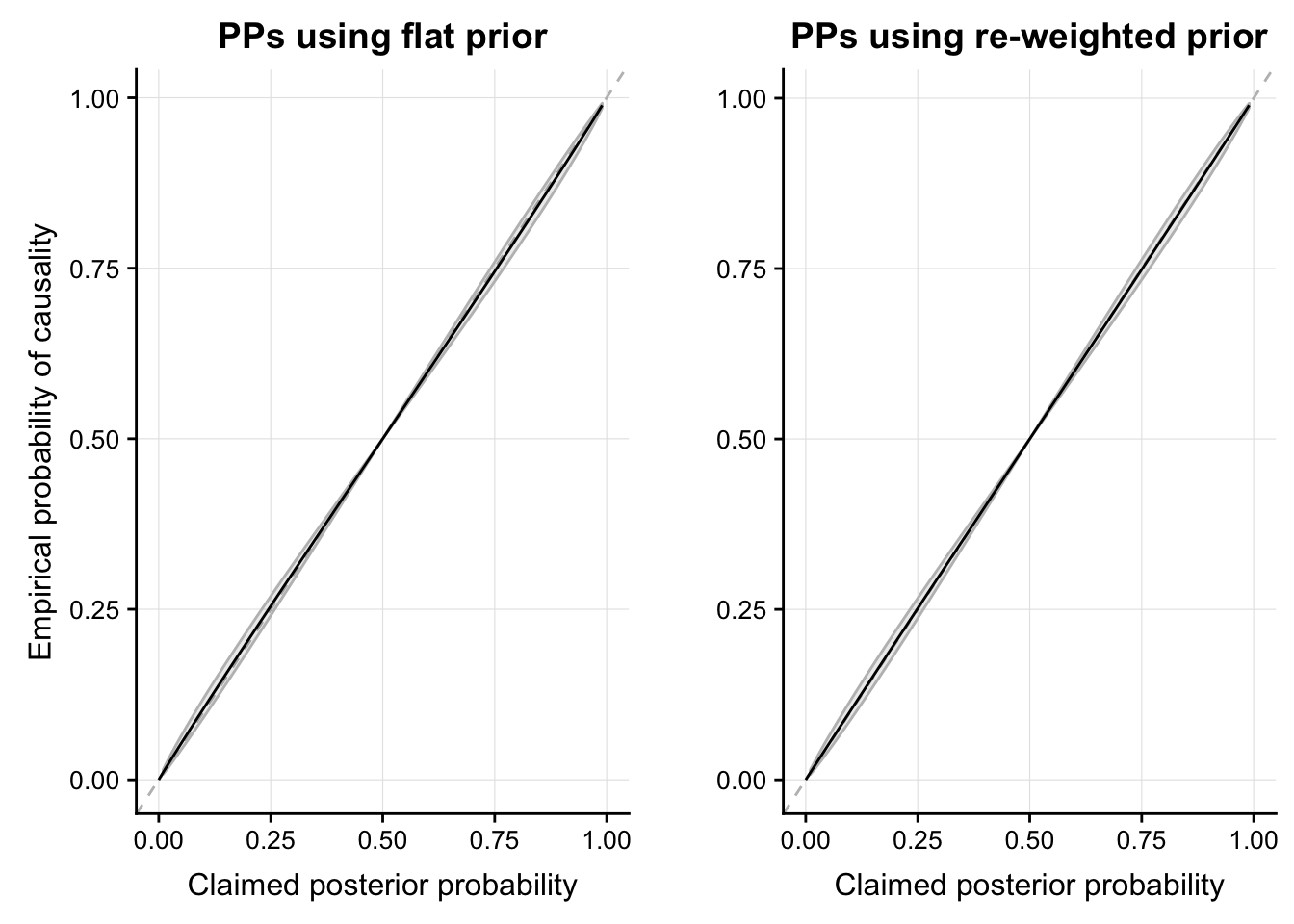We hypothesise that the flat prior used in the single causal variant fine-mapping method to obtain credible sets of putative causal variants could be contributing to the coverage bias. After the variants have been ranked in descending order of posterior probability of causality (PPs), we can afford to be “more sure” that the variants are the front of the ordering are more likely to be causal than the variants at the back of the ordering (given that their PPs vary substantially), however this extra information is not utilised in the procedure. We investigate whether we can incorporate this extra information into the prior, and use this with the approximate Bayes factors (ABFs) to derive the PPs.

#### Dirichlet distribution

The support for a dirichlet distribution is $x_1,...,x_K \text{ where } x_i \in (0,1) \text{ and} \sum_{i=1}^K x_i = 1.$ I.e. $$K$$ probabilities which sum to 1 - so it is a distribution on probability distributions which is often used as a prior in Bayesian statistics.

The pdf for $$\theta \sim Dir(\alpha)$$ is, $p(\theta)=\frac{1}{\beta(\alpha)}\Pi_{i=1}^n \theta_i^{\alpha_i-1} I(\theta \in S)$ where $$S$$ is the probability simplex. So that if $$\alpha=1$$ then the pdf is constant and is the uniform distribution over the probability simplex.

# https://www.youtube.com/watch?v=nfBNOWv1pgE

##' Function to generate random deviates from the Dirichlet distribution.
##'
##' @title
##' @param n number of random vectors to generate
##' @param a vector containing shape parameters
##' @return
rdirichlet<-function(n, a){
l <- length(a);
x <- matrix(rgamma(l*n,a),ncol=l,byrow=TRUE); # generate l*n rgamma random quantities
sm <- x %*% rep(1, l); # calculate row sums
x/as.vector(sm); # normalise to sum to one
}

x <- rdirichlet(10000, c(1,1,1))
colMeans(x) # will tend to 1/3 each
##  0.3292669 0.3348472 0.3358859
x <- rdirichlet(10000, c(1,1,8))
colMeans(x) # x1 and x2 approx 1/10, x2 approx 1/8
##  0.09960085 0.10045153 0.79994762

The standard prior used in single causal variant fine-mapping is flat and so we put a probability distribution on this and choose the dirichlet distribution with $$\alpha=\bf{1}$$. Note that the flat prior used is the expectation of this dirichlet distribution.

If we use a random vector generated from this dirichlet distribution as the prior, we can take a sampled value from this and see whether or not this is the CV.

##' Sample one SNP from pi (realisation from dirichlet, i.e. prior on all one snp models)
##'
##' Return TRUE is the CV is chosen
##' @title
##' @param nsnps number of snps
##' @param iCV index of CV
##' @return
draw <- function(nsnps, iCV = 1){
pi <- rdirichlet(1, rep(1, nsnps))
sample(nsnps, 1, prob = pi) == iCV
}

draw(100)
##  FALSE
# MC estimate of prob of drawing CV (SNP 1) under prior
# probabilities that each SNP is picked
mean(sapply(1:10000, function(i) draw(100)))
##  0.0088

# IS THE SNP CHOSEN FROM THE PRIOR PROB VECTOR THE ONE WITH THE MAX PROB?

##' Draw hyper copy of pi, sample 1 value
##' return TRUE if that was the SNP with max pi value
##'
##' @title
##' @param nsnps number of snps
##' @param hyper hyper-parameter for alpha
##' @return
draw_top <- function(nsnps, hyper = 1){
pi <- rdirichlet(1, rep(hyper, nsnps))
sample(nsnps, 1, prob = pi)==which.max(pi)
}

draw_top(100)
##  FALSE
# IS THE SNP CHOSEN FROM N DIFFERENT PRIOR PROB VECTORS THE ONE WITH THE MAX PROB?

##' Draw hyper copy of pi, sample 1 value
##' return vector of TRUE/FALSE for whether
##' the sampled SNP was the one with the
##' max pi value
##'
##' @title
##' @param nsnps number of snps
##' @param n number of random vectors to generate
##' @param hyper hyper-parameter for alpha
##' @return
draw_top <- function(nsnps, n = 1, hyper = 1){
pi <- rdirichlet(n, rep(hyper, nsnps))
sapply(1:n, function(i)
sample(nsnps, 1, prob = pi[i,])==which.max(pi[i,]))
}

draw_top(2, n = 5)
##  TRUE TRUE TRUE TRUE TRUE
draw_top(100, n = 5)
##  FALSE FALSE FALSE FALSE FALSE
# MC estimate of drawing top model under prior
mean(sapply(1:10000, function(i) draw_top(50)))
##  0.0919

# ORDER SNPS IN DESCENDING ORDER OF PRIOR PROBS AND SEE WHAT POSITION THE
# SAMPLED SNP IS IN
# expect this to be near the front

##' Draw hyper copy of pi, sample 1 value
##' return 1 at the position of the sampled SNPs ordering
##'
##' @title
##' @param nsnps number of snps
##' @param hyper hyper-parameter for alpha
##' @return
draw_order <- function(nsnps, hyper=1){
pi <- rdirichlet(1, rep(hyper, nsnps))
draws <- sample(nsnps, 1, prob = pi)
sapply(order(pi, decreasing = TRUE), function(x) sum(x == draws)) # the sum bit just changes TRUE/FALSE to binary
}

draw_order(nsnps = 100)
##    0 0 0 0 0 0 0 0 0 0 0 0 0 0 0 0 0 0 1 0 0 0 0 0 0 0 0 0 0 0 0 0 0 0 0
##   0 0 0 0 0 0 0 0 0 0 0 0 0 0 0 0 0 0 0 0 0 0 0 0 0 0 0 0 0 0 0 0 0 0 0
##   0 0 0 0 0 0 0 0 0 0 0 0 0 0 0 0 0 0 0 0 0 0 0 0 0 0 0 0 0 0

#### MC estimates

# MC estimate of prob of drawing 1st model under prior
mean(sapply(1:10000, function(i) draw(50, iCV  = 1))) 
##  0.0196
# 50 models: take 10000 draws and average how often the chosen SNP is the CV (SNP 1)

# MC estimate of drawing top model under prior
mean(sapply(1:10000, function(i) draw_top(50))) 
##  0.0897
# 50 models: take 10000 draws and average how often the chosen SNP is the top SNP (highest prior)

# MC estimate of drawing each ordered variant
rowMeans(sapply(1:10000, function(i) draw_order(50, 1))) 
##   0.0838 0.0718 0.0591 0.0512 0.0516 0.0442 0.0414 0.0358 0.0372 0.0328
##  0.0308 0.0318 0.0305 0.0284 0.0252 0.0237 0.0206 0.0194 0.0194 0.0184
##  0.0192 0.0175 0.0177 0.0160 0.0139 0.0128 0.0131 0.0121 0.0116 0.0099
##  0.0103 0.0098 0.0090 0.0086 0.0064 0.0076 0.0065 0.0083 0.0056 0.0057
##  0.0041 0.0025 0.0034 0.0026 0.0031 0.0025 0.0013 0.0009 0.0004 0.0005
# 50 models: take 10000 samples, what probabilities are the ordered variants picked

### Generalise to our method (walkthrough example)

We want to use pi_new as our new prior that a variant in an ordered vector of ABFs is causal.

pi_new <- rowMeans(sapply(1:10000, function(i) draw_order(50, 1))) 

An example of this new ordered prior vector is plotted below, with the original prior plotted in red.The PPs can now be calcuated,

# calculate abfs
W = 0.2
r = W^2/(W^2 + varbeta)
labf = 0.5 * (log(1 - r) + (r * z0^2))
abf = exp(labf)

# calculate ordered pps
o = order(abf,decreasing=TRUE)
ordered_pp = abf[o]*pi_new/sum(abf[o]*pi_new)

Relative to the original PPs, the new PPs for SNPs nearer to the front of the ordering will be bigger and the PPs for the SNPs nearer the back of the ordering will be smaller (since they are given bigger and smaller priors respectively).

Continuing with the example, below the black points are the re-weighted PPs and the red points are the original PPs - the reweighting method “stretches out” the distribution of PPs.### Empirical calibration

We next want to investigate whether these re-weighted PPs are calibrated.

To investigate this, we plot logit(cv)~logit(pp) where cv is a binary indicator of whether that SNP is the CV and pp are the corresponding PPs (exactly how we derive Fig. S2 in the paper).

The following plots are for 200 simulated PP systems. It seems that the re-weighted PPs are also well empirically calibrated.### 1. Empirical calibration of top SNP

Let’s focus on the empirical calibration of the variant with the highest PP.

To investigate this, I store what position the top SNP’s PP is in the system to correct and average the 5000 simulated PP values at this SNP to assess acccuracy. E.g. if the top SNP is at position 15 then I average the simulated PPs for SNP 15 to get the empirical PP of this top SNP.

#### Flat Prior

• The y axis is the top PP in the system to correct (top PP) minus the empirically calculated PP for this SNP (empirical PP).

• Does this make sence? It seems that the empirically estimated PP is consistently too low and that $$top PP>empirical PP$$.